# Synthetic division

In Mathematics, there are two different methods to divide the polynomials. One is the long division method. Another one is the synthetic division method. Among these two methods, the shortcut method to divide polynomials is the synthetic division method. It is also called the polynomial division method of a special case when it is dividing by the linear factor.  It replaces the long division method.  In certain situations, you can find this method easier. In this article, we will discuss what the synthetic division method is, how to perform this method, steps with more solved examples.

## Synthetic Division of Polynomials

The Synthetic division is a shortcut way of polynomial division, especially if we need to divide it by a linear factor. It is generally used to find out the zeroes or roots of polynomials and not for the division of factors. Thus, the formal definition of synthetic division is given as:

“Synthetic division can be defined as a simplified way of dividing a polynomial with another polynomial equation of degree 1 and is generally used to find the zeroes of polynomials”

This division method is performed manually with less effort of calculation than the long division method. Usually, a binomial term is used as a divisor in this method, such as x – b.

If we divide a polynomial P(x) by a linear factor (x-a), which of the polynomial of the degree 1, Q(x) is quotient polynomial and R is the remainder, which is a constant term. We use the synthetic division method in the context of the evaluation of the polynomial using the remainder theorem, wherein we evaluate the polynomial P(x) at “a” while dividing the polynomial P(x) by the linear factor. (i.e) P(x)/(x-a).

Mathematically, it can be represented as follows:

P(x)/Q(x) = P(x)/(x-a) = Quotient + [Remainder/(x-a)]

(i.e)

P(x)/(x-a) = Q(x) +[R/(x-a)]

Hence, we can use the synthetic division method to find the remainder quickly, if “a” is the factor of the polynomial.

In the synthetic division method, we use only the numbers for calculation and this method avoids the usage of the variables.

Note:

• We can perform the synthetic division method, only if the divisor is a linear factor.
• In the synthetic division method, we will perform multiplication and addition, in the place of division and subtraction, which is used in the long division method.

## How to Perform a Synthetic Division?

If we want to divide polynomials using synthetic division, you should be dividing it by a linear expression and the first number or the leading coefficient should be a 1. This division by linear denominator is also called division through Ruffini’s rule(paper-and-pencil computation).

The requirements to perform the synthetic process method is given below:

• The divisor of the given polynomial should be of degree 1. It means that the exponent of the given variable should be 1. Such kind of divisor is considered as the linear factor.
• The coefficient of the divisor variable (say x) should be also equal to 1.

The process of the synthetic division will get messed up if the divisor of the leading coefficient is other than one. In case if the leading coefficient of the divisor is other than 1 while performing the synthetic division method, solve the problem carefully.

The basic Mantra to perform the synthetic division process is”

For example, we can use the synthetic division method to divide a polynomial of 2 degrees by x + a or x – a, but you cannot use this method to divide by x2 + 3 or 5x2 – x + 7.

If the leading coefficient is not 1, then we need to divide by the leading coefficient to turn the leading coefficient into 1. For example, 4x – 1 would become x – ¼ and 4x+9 would become x + 9/4. If the synthetic division is not working, then we need to use long division.

## Steps for Polynomial Synthetic Division Method

Following are the steps required for Synthetic Division of a Polynomial:

 Step 1 To set up the problem, we need to set the denominator = zero, to find the number to put in the division box. Then, the numerator is written in descending order and if any terms are missing we need to use a zero to fill in the missing term. At last, list only the coefficient in the division problem. Step 2 Now, when the problem is set up perfectly, bring the first number or the leading coefficient straight down. Step 3 Then, put the result in the next column by multiplying the number in the division box with the number you brought down. Step 4 Write the result at the bottom of the row by adding the two numbers together Step 5 Until you reach the end of the problem, repeat steps 3 and 4. Step 6 Write the final answer. The numbers in the bottom row with the last number being the remainder and the remainder which is written as a fraction makes the final answer. The variables shall start with one power less than the real denominator and go down one with each term.

The advantages of using the synthetic division method are:

• It requires only a few calculation steps
• The calculation can be performed without variables
• Unlike the polynomial long division method, this method is a less error-prone method

The only disadvantage of the synthetic division method is that this method is only applicable if the divisor of the polynomial expression is a linear factor.

### Synthetic Division Examples

Example 1:

$$\begin{array}{l}\text{Divide: }\frac{2x^{3} – 5x^{2} + 3x + 7}{x-2}\end{array}$$

Solution:

Following the steps as per explained above, to divide the polynomials given. Thus, we can get;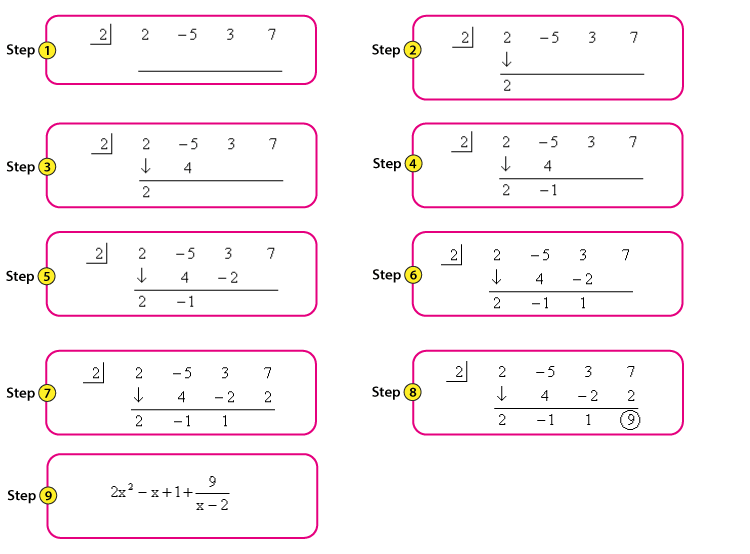Synthetic Division Example 1

Example 2:

$$\begin{array}{l}\text{Divide: }\frac{2x^{3} + 5x^{2} + 9}{x+3}\end{array}$$

Solution:

As per the given question; we have two polynomials in numerator and denominator. The denominator consists of a linear equation, so we can easily apply the synthetic division method here.

Follow the step by step method as given below: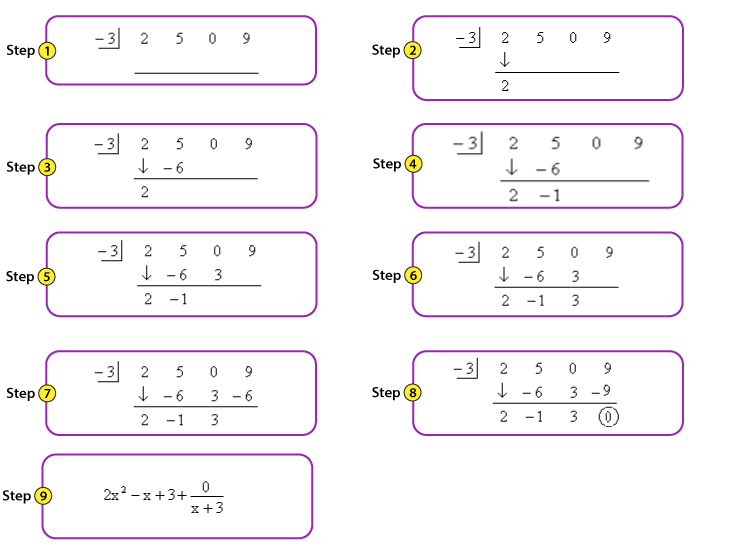Example 3:

$$\begin{array}{l}\text{Divide: }\frac{3x^{3} + 5x – 1}{x+1}\end{array}$$

Solution:

Following the same steps as per previous examples.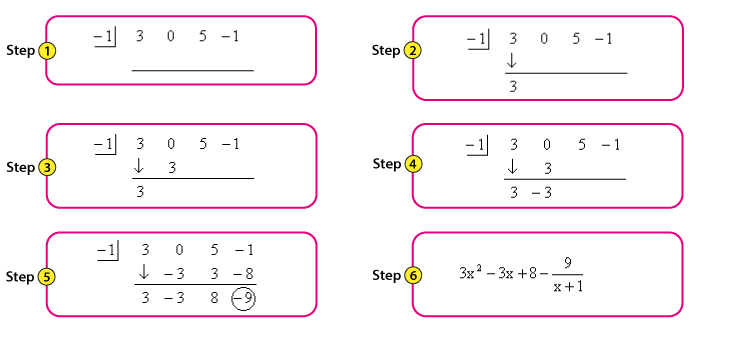Synthetic Division Example 3

Example 4:

$$\begin{array}{l}\text{Divide: }\frac{4x^{3} – 8x^{2} -x +5}{2x – 1}\end{array}$$

Solution:

As we know, the step to solve the given equation by synthetic division method, we can write;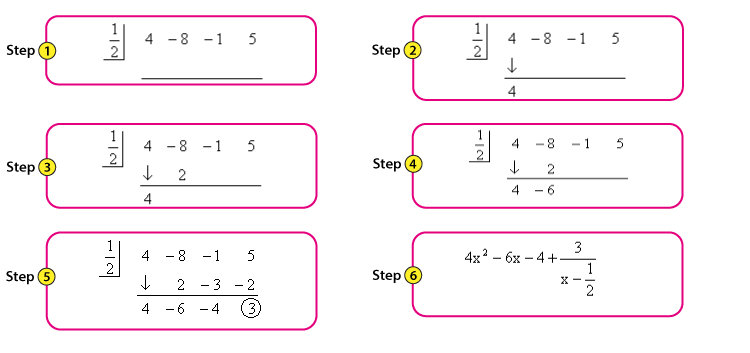Now, divide the answer obtained in step 6 by 2. Hence, we get

$$\begin{array}{l}=\frac{4x^2 – 6x – 4 + \frac{3}{x – \frac{1}{2}}}{2}\end{array}$$
$$\begin{array}{l}=2x^2 – 3x – 2 + \frac{3}{2x – 1}\end{array}$$

Example 5:

$$\begin{array}{l}\text{Divide: }\frac{x^{3} – 5x^{2} +3x + 7}{x – 3}\end{array}$$

Solution:

Solving the given expression, by step by step method, we get;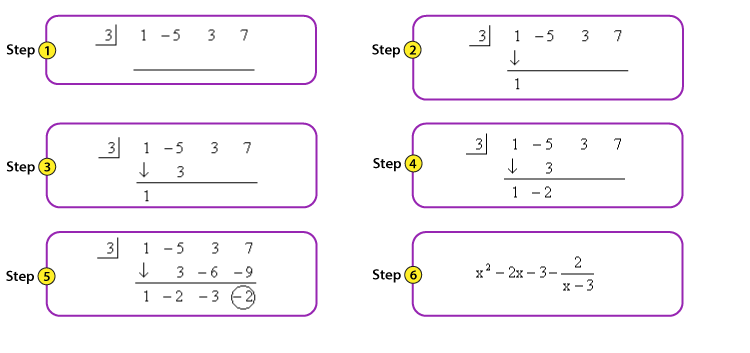### Synthetic Division of polynomials Practice Questions

Solve the following problems:

1. Find the quotient and remainder of the polynomial 2x3 -7x2 +0x+11, when it is divided by a linear factor x-3.
2. Solve the following polynomial equation and find its quotient and remainder. (9a2-39a-30)/(a-5)
3. Find Q(x) and R for the polynomial, P(x)=m3-3m+4 divided by the linear factor m-1.

## Frequently Asked Questions on Synthetic Division

Q1

### What is meant by synthetic division?

The synthetic division method is a special method of dividing polynomials. This method is a special case of dividing a polynomial expression by a linear factor, in which the leading coefficient should be equal to 1.

Q2

### What are the requirements of the synthetic division method?

The requirements of the synthetic division method are:
The divisor of the polynomial expression must have a degree of one (linear factor)
The leading coefficient of the variable in the divisor should be equal to 1.

Q3

### What is the Main Use of Synthetic Division?

Synthetic division is mainly used to find the zeroes of roots of polynomials.

Q4

### When Can You Use Synthetic Division?

Synthetic division is used when a polynomial is to be divided by a linear expression and the leading coefficient (first number) must be a 1. For example, any polynomial equation of any degree can be divided by x + 1 but not by x2+1

Q5

### Why is Synthetic Division Important?

Synthetic division is useful to divide polynomials in an easy and simple way as it breaks down complex equations into smaller and easier equations.